Quantity: 0

Total: 0,00

0

# Perimeter, area, surface area and volume

### Perimeter, area, surface area and volume

This animation presents the formulas to calculate the perimeter and area of shapes as well as the surface area and volume of solids.

Mathematics

Keywords

volume, surface, circumference, area, sphere, pyramid, cylinder, circle sector, circle, triangle, rectangle, square, cone, cuboid, base area, lateral surface, parallelogram, formula, geometry, solid geometry, mathematics

Related items

### Scenes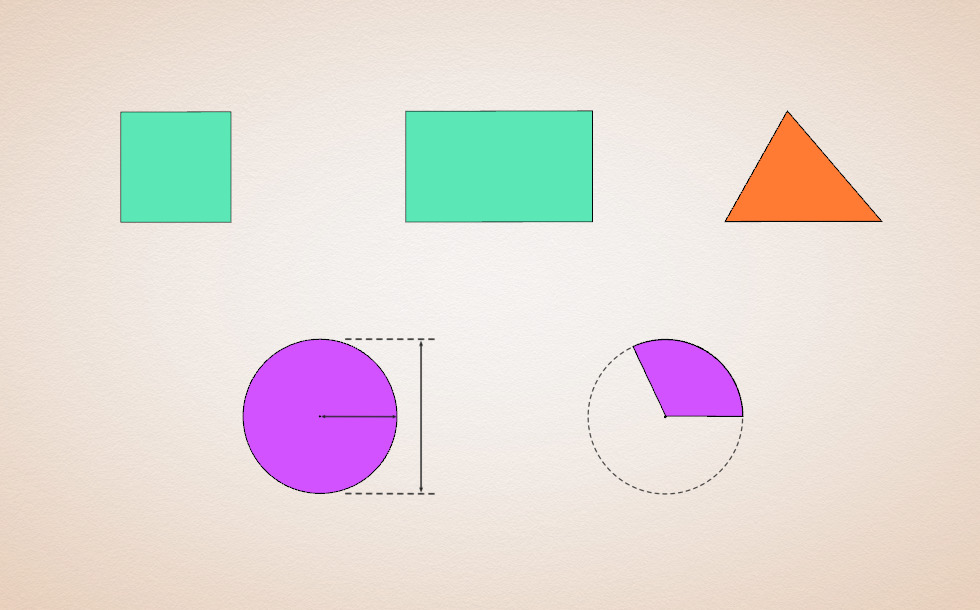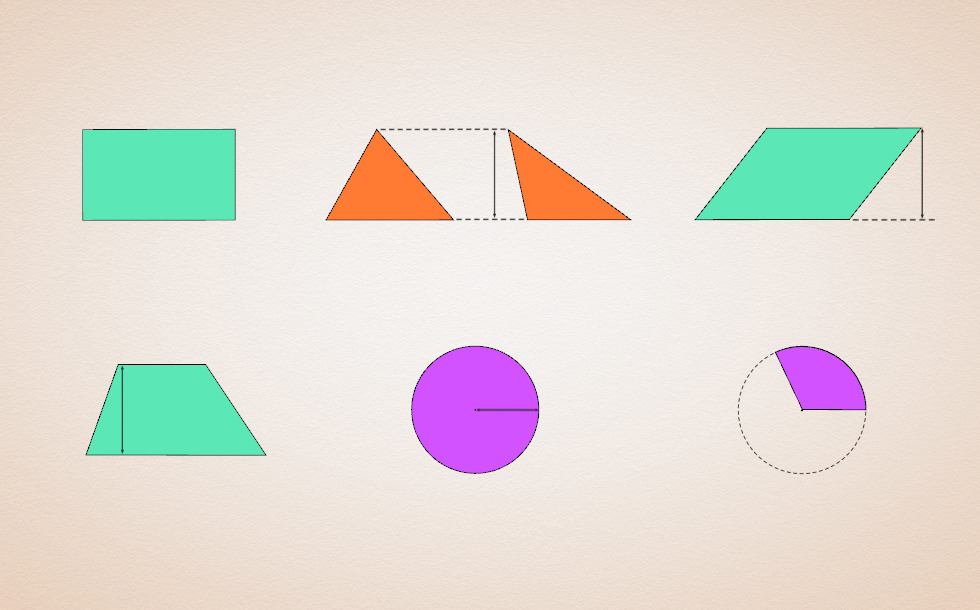• trapezoid

A two-dimensional geometric shape is a part of a plane that is enclosed by straight or curved lines, does not contain any holes and remains intact even if one of its points is removed.

The area is a function that assigns a positive number to all two-dimensional geometric shapes with the following conditions:

1. The area of the unit square is 1.
2. The area of congruent geometric shapes is equal.
3. If we divide a geometric shape into several parts, the sum of the areas of the parts is equal to the area of the original geometric shape.

The area of a rectangle is the product of its width and height.

The area of a triangle is half the product of its base length and its height. (This formula derives from the formula of the area of the parallelogram.)

The area of a parallelogram is the product of its base length and height.

The area of a trapezoid is the product of half the sum of the parallel sides and its height.

The area of a circle can be calculated by multiplying the square of its radius with π (pi).

The area of a circular sector can be calculated from the area of the full circle, using the ratio of the central angle to the full angle (360°).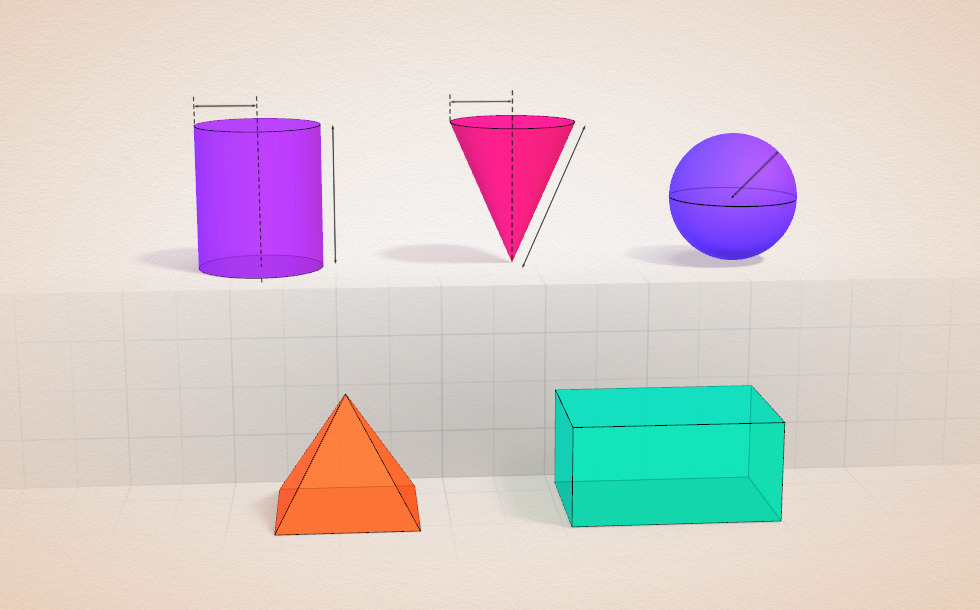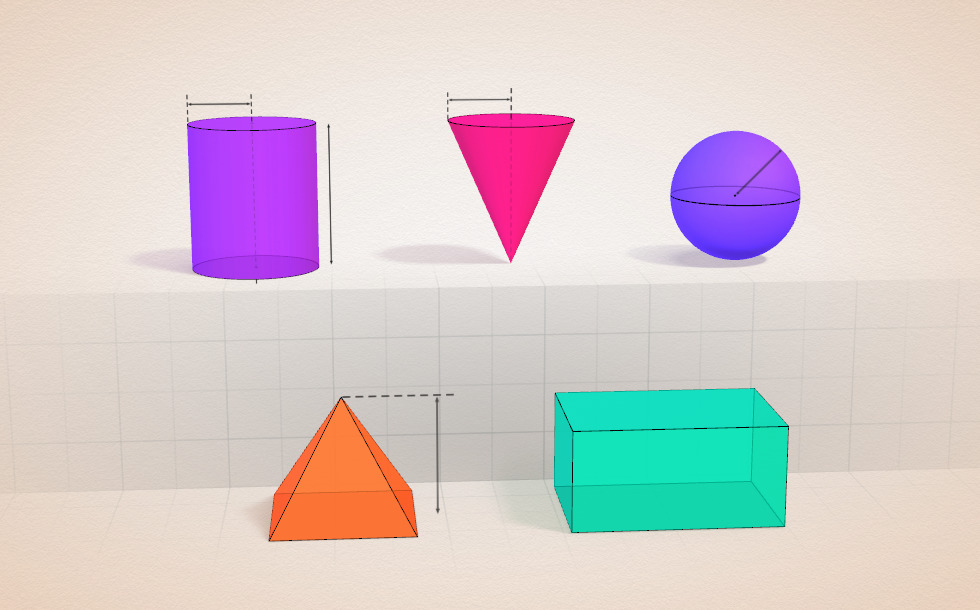### Related items#### Cuboid

A cuboid is a polyhedron with six rectangular faces.#### Conic solids

This animation demonstrates various types of cones and pyramids.#### Cube

This animation demonstrates the components (vertices, edges, diagonals and faces) of the cube, one of the Platonic solids.#### Sphere

A sphere is the set of points which are all within the same distance from a given point in space.#### Conic sections

The conic section is a plane curve that is created when a right circular cone is intersected by a plane.#### Grouping of cuboids

This animation demonstrates various types of cuboids through everyday objects.#### Platonic solids

This animation demonstrates the five regular three-dimensional (or Platonic) solids, the best known of which is the cube.#### Ratio of volumes of similar solids

This 3D scene explains the correlation between the ratio of similarity and the ratio of volume of geometric solids.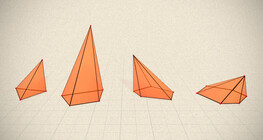#### Regular square pyramid

A regular square pyramid is a right pyramid with a square base and four triangular faces.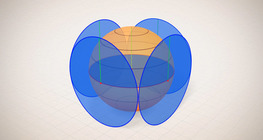#### Surface area of spheres (demonstration)

The surface of a sphere consists of the set of points which are all at the same distance from a given point in space.#### Volume and surface area (exercise)

An exercise about the volume and surface area of solids generated from a ´base cube´.#### Volume of spheres (Cavalieri´s principle)

Calculating the volume of a sphere is possible using an appropriate cylinder and cone.#### Volume of spheres (demonstration)

The sum of the volume of the ´tetrahedrons´ gives an approximation of the volume of the sphere.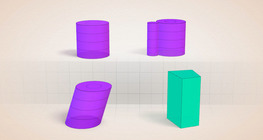#### Cylindrical solids

This animation demonstrates various types of cylindrical solids as well as their lateral surfaces.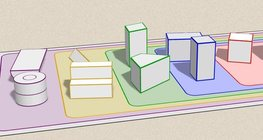#### Grouping of solids

This animation demonstrates various groups of solids through examples.#### Net of a cube (exercises)

Not all nets consisting of 6 congruent squares are foldable into cubes.#### Solids of revolution

Rotating a geometric shape around a line within its geometric plane as an axis results in a solid of revolution.